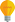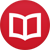# Is it possible to divide by zero?

by Jakub MarianTip: See my list of theMost Common Mistakes in English. It will teach you how to avoid mis­takes with com­mas, pre­pos­i­tions, ir­reg­u­lar verbs, and much more.

This is a common question on mathematical discussion boards, with people sometimes feeling that mathematicians are too closed-minded for not wanting to consider the poster’s brilliant idea about how it is possible to divide by zero.

Such an opinion stems from a misunderstanding of what mathematics really is. In mathematics, we don’t discover mathematical objects. Instead, we define objects and study their properties. Hence, assertions like “$1/0$ must be $∞$ because …” don’t really make sense; $1/0$ either is something by the definition or it is left undefined by it.

Formally, division is just a function of two variables. You can imagine it like a huge (actually infinite) table looking like

 / 1 2 3 … 1 1 0.5 0.333… … 2 2 1 0.666… … 3 3 1.5 1 … … … … … …

When you want to know what $3/2$ is, you go to the “third” row and “second” column, and you see that it is $1.5$. It is impossible to write down the entire table, as it is (uncountably) infinite and contains all numbers like $10.561234$ or $π$. What mathematicians can do, however, is to write down a set of rules that describe what each cell of the table looks like.

As it happens, the table, as mathematicians have defined it, doesn’t contain a column for $0$. We say that $1/0$ is undefined because there is no corresponding cell for it. $1/0$ is undefined in the same way as ${+}/{*}$ or ${∫}/2$ is undefined.

## Is it useful to define division by zero?

That’s the correct question to ask. Since we define, not discover, what $a/b$ means, we can ask: Can we define $a/0$ in a way that is useful?

The most common reason to define expressions like $a/0$ is to shorten the notation in some theory. For example, when computing limits of positive sequences, it may be useful to define $a/0 = ∞$ for $a ≠ 0$ so that we may conveniently write things like

$$\lim_{n→∞} ÷{1+÷{1}{n}}{e^{-n}} = ÷{\lim_{n→∞}(1+÷{1}{n})}{\lim_{n→∞}e^{-n}} = ÷{1}{0} = ∞\,.$$

The reason to define it like that in this context is that it shortens calculations. In other theories, other strange-looking shorthands are used; it is common to write $0⋅∞ = 0$ in measure theory (even though it wouldn’t make sense in the theory of limits) since it, yet again, simplifies notation:

$$∫_ℝ 0\, dλ = 0⋅λ(ℝ) = 0⋅∞ = 0\,.$$

However, when speaking about division by zero, people usually imagine some form of arithmetics, like $1/0 = ∞$, so $5/0=5⋅∞$, and so on. This is partially done in complex analysis when we work with the so called Riemann sphere which is just $ℂ ∪ \{∞\}$. For various reasons, it is practical to define

$$÷{z}{0} = ∞$$

for $z ∈ ℂ \setminus \{0\}$. Nonetheless, this operation is not reversible, i.e. it is not possible to write something like $÷{z}{0}⋅0 = z$.

It is actually possible in principle to construct an arithmetics which, to a certain degree, extends the traditional one and in which writing $1/0 = ∞$ makes sense, but in order to do that, it is necessary to sacrifice some algebraical properties. But this is the topic of the next article.

By the way, I have written several educational ebooks. If you get a copy, you can learn new things and support this website at the same time—why don’t you check them out?